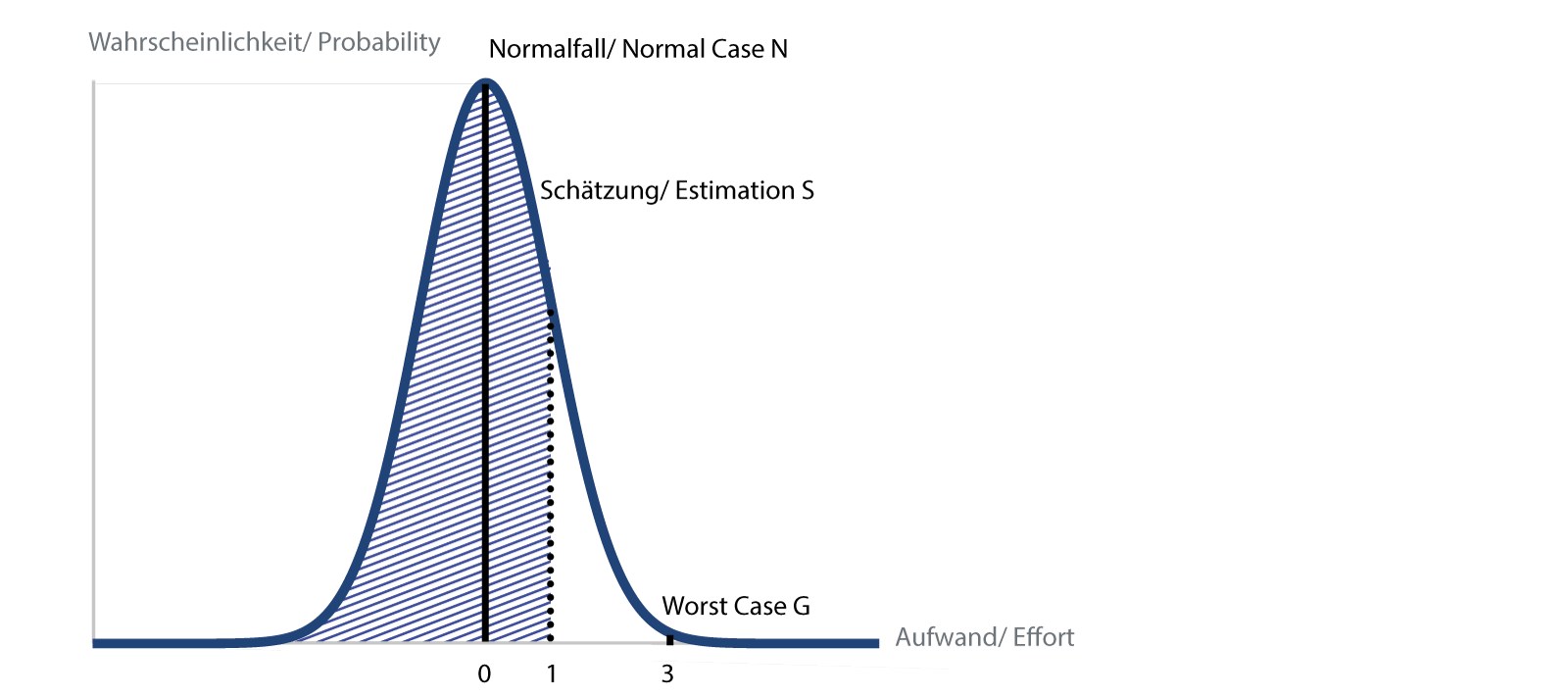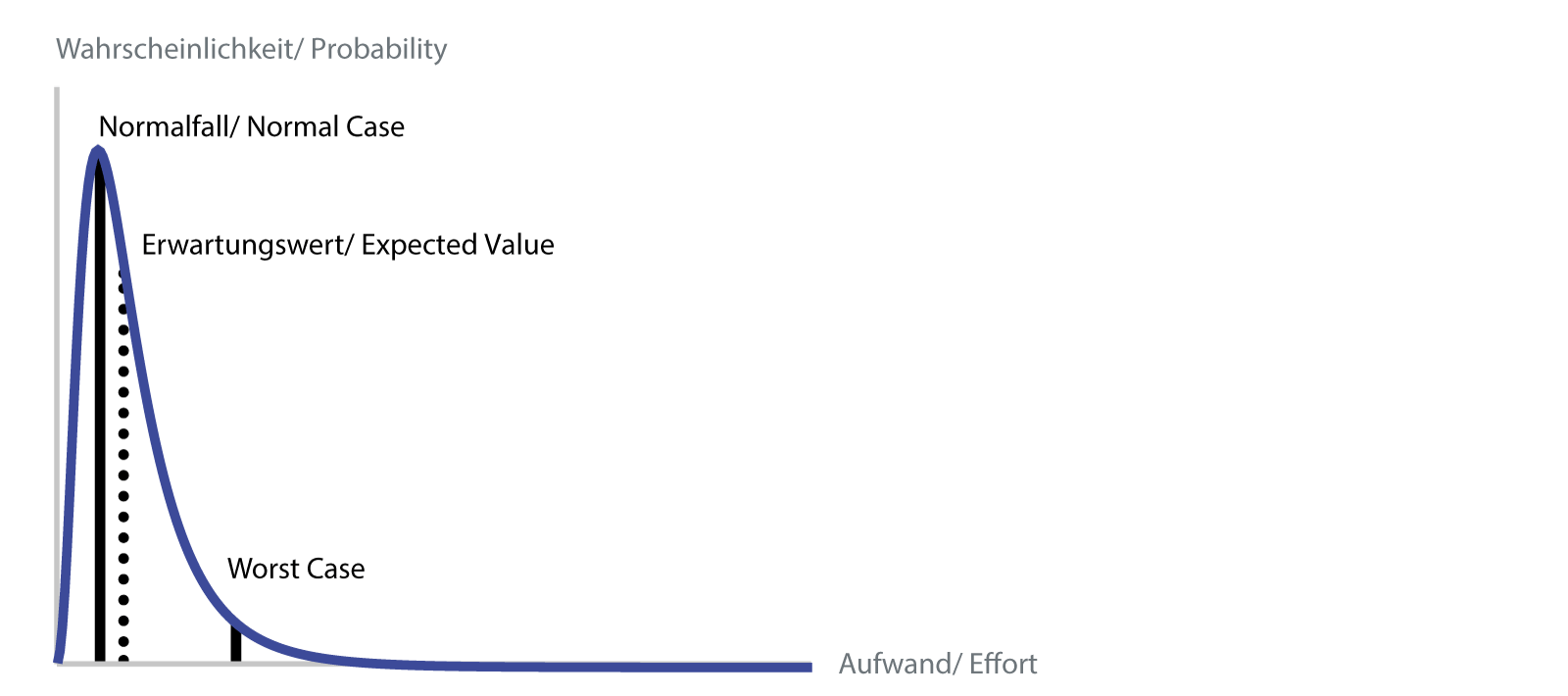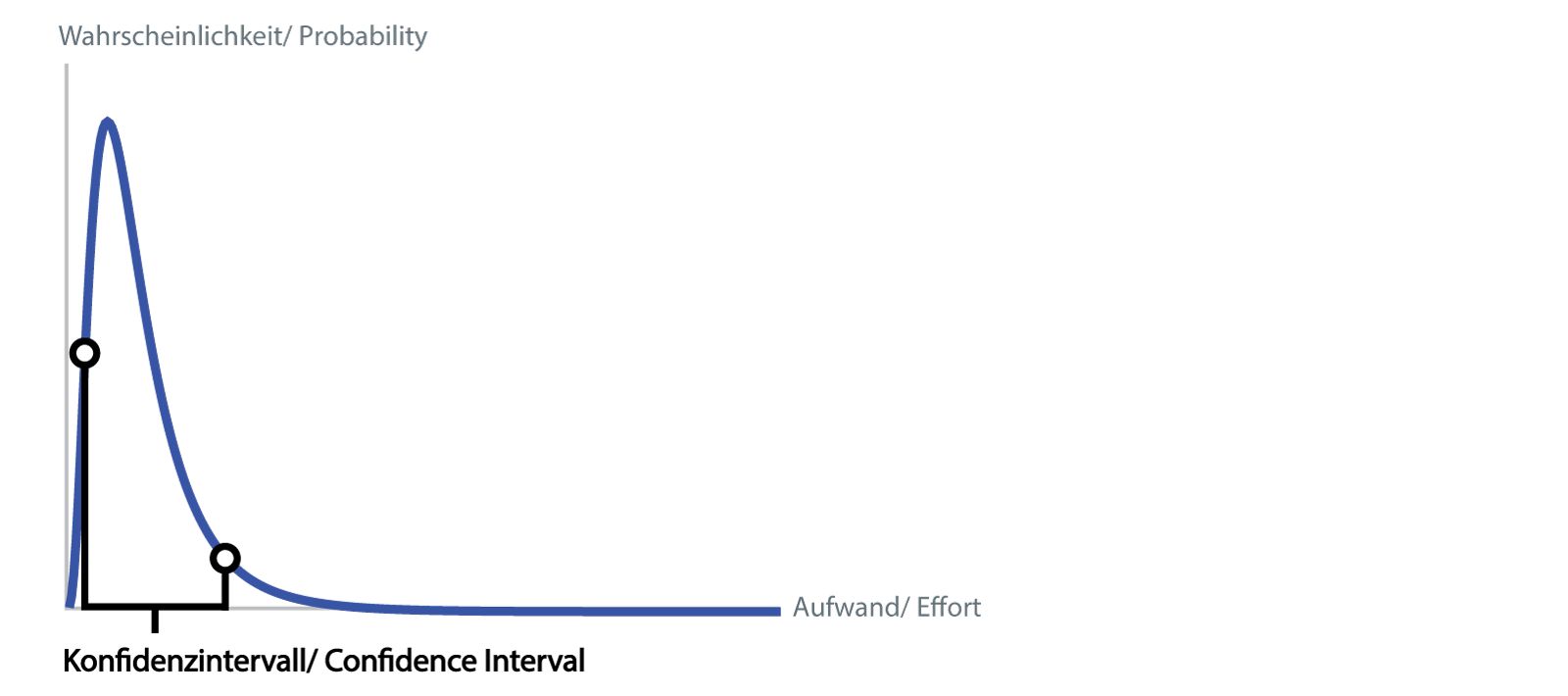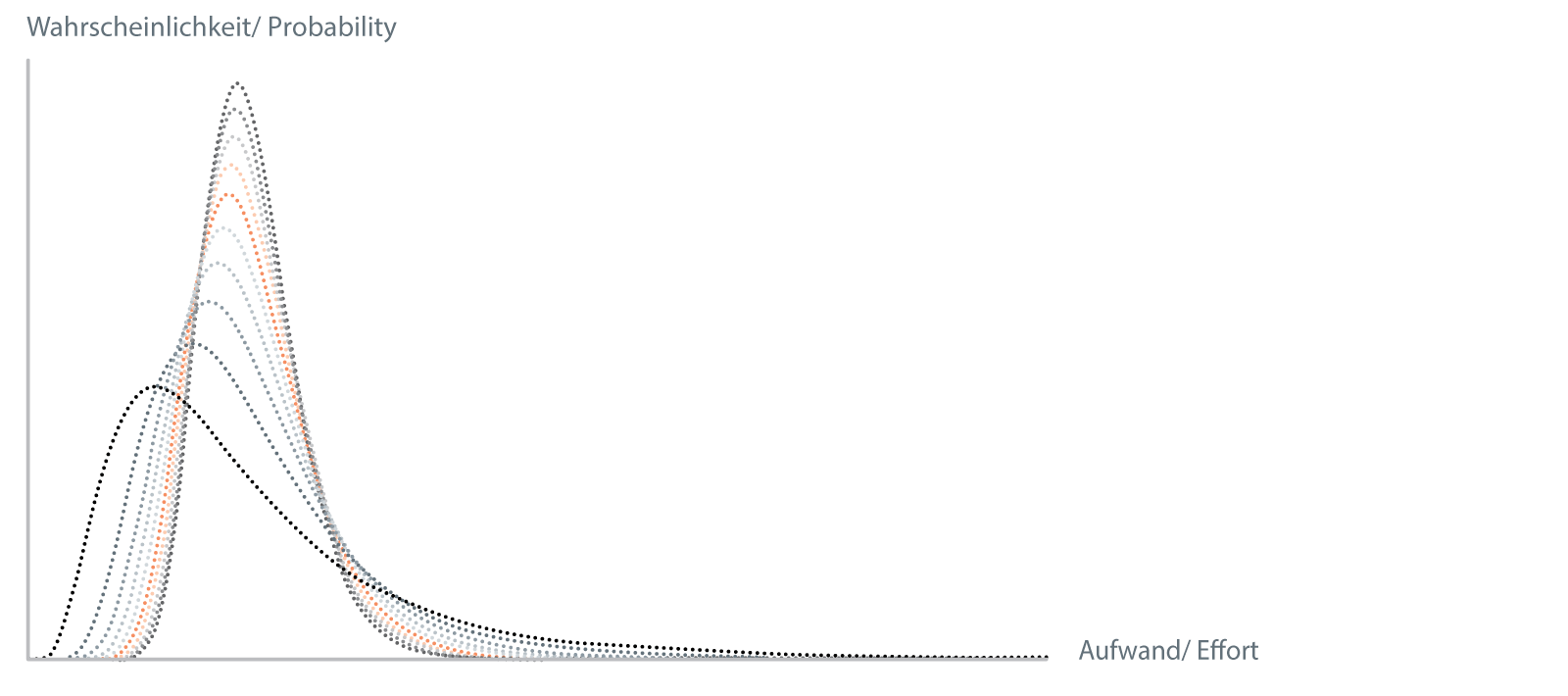# How to Deal with Fuzziness? Distributions!

"All models are wrong, but some are useful" G. Box

It is not possible to estimate exactly. Otherwise it would not be an estimate... So how do I deal with the fact that I am not sure whether I need 20 hours or perhaps 40 for a task? Mathematics gives us tools for this, namely probability distributions, which can serve as a model.

In the probability distribution below, the horizontal axis shows the effort in hours, and the vertical axis shows the probability that these hours will be used.Probability Distribution (lognormal)

Now, of course, one does not want to discuss the exact curve for each work package, so one assumes certain curve shapes as a model that can be described with a few parameters.

In the next sections, different approaches to this will be discussed.

## Three-Point Effort Estimation

In a revival of "scientific management" in the 1950s, the beta distribution was used for PERT (Program Evaluation and Review Technique) [stutzke]. The estimators must estimate three values (hence 3-point effort estimation):

• Normal case N: most likely effort (mode)
• Optimal case K: smallest possible effort
• Worst case G: largest possible effort

Using the beta distribution, a fairly simple formula is obtained to calculate the estimated value S (expected value: the effort that is needed on average):Experience has shown that there is often a tendency to optimism among estimators as tasks and difficulties are forgotten. Therefore, Barry W. Boehm (in [R. D. Stutzke: «Estimating Software Intensive Systems», 2005, ISBN 0-201-70312-2]) has given greater weight to the worst case value G in the 3-point effort estimation by shifting a weight from the normal case to the worst case:## Two-Point Estimation

Indirectly, [W. Reiter: «Die nackte Wahrheit über Projektmanagement», 2003 ISBN 3-280-05018-9] chose a different approach by shifting the weight from the optimal case K to the worst case G in his formula:In addition to the optimism correction, this two-point effort estimation has the advantage that one of three estimation values and thus a part of the estimation effort can be saved.

### Optimism? Really?

In [N. N. Taleb: «Antifragile», 2012 ISBN 1-400-06782-0], in the chapter "Why planes don't arrive early" there is another, philosophical explanation for the fact that projects always tend to be underestimated. One rarely plans too much, the more complex the project, the activity, the more is forgotten. Since the time spent on such forgotten activities is always positive, the total effort can only increase, never decrease.

### Justification of the two-point effort estimation with the normal distribution

If the whole PERT story is too empirical for you, you can also justify the above formula with the normal distribution, as in [W. Reiter: «Die nackte Wahrheit über Projektmanagement», 2003 ISBN 3-280-05018-9], according to the following illustration:## Two-Point Estimation

Normal distribution with estimate and the 84% area marked

Marked are the normal case N and the worst case G. Now we specify that we want to be below the worst case G for 99.9% of the cases. This allows us to fit a normal distribution ("Gaussian curve/ Bell curve"), where the normal case corresponds to the peak value, i.e. the most frequent case, the worst case corresponds to a deviation of three standard deviations ("3 sigma"), the small remainder above then corresponds to a probability of 0.1 %.

We further specify that we would like our estimate to be below the estimated value in 84 % of the cases. This corresponds to a standard deviation ("1 sigma"), which is on the third of the way between normal and worst case. Written out as a formula:So the same result as above. What is unattractive about this type of reasoning is the fact that the normal distribution also allows for negative effort. While this would sometimes be nice for project managers, I have not heard of anyone who has finished a job before they started.

## Lognormal Distribution

This inelegance, namely that negative effort is possible, can be prevented with another distribution, the lognormal distribution which starts at zero. In addition, the asymmetry of the curve better represents the reality of the development [S. McConell: «Software Estimation: Demystifying the Black Art», 2006 ISBN 978-0-7356-0535-0].Lognormal distribution with estimated values

As a model for our purposes the lognormal distribution is suitable, because on the one hand it covers a range of values above zero and on the other hand it can be completely characterized by two parameters, in our case by the normal value and the worst case. The normal case is defined as the case where the probability is greatest, that is, the peak value. The worst case value can again be chosen at 99.9%. We ourselves use 95%, this also results in a certain correction of the optimism of the estimators.

The expected value, i.e. the value that one would most likely expect, is the mean value, which in this distribution, unlike the normal distribution, does not coincide with the peak value. The big disadvantage of the lognormal distribution is that one has to use numerical methods or Monte Carlo simulations to calculate sums and products. A simple implementation as a spreadsheet is not possible.

## The 90% Confidence Interval: How much Risk would You Like to take?

Once you have calculated the probability distribution of the entire project, not only can a value be extracted, but a range can be specified for the estimate. We use the 90% confidence interval, i.e. with 90% probability the real effort (under the assumptions made i.e. excluding the separate risks of the risk list) lies within the range.Confidence Interval

In other words, the probability is 5% that the effort is below the lower threshold, 5% that it is above the upper threshold. The width of the confidence interval also provides a good measure of the risk of the project: an interval of 2000 to 6000 hours indicates a riskier project than one of 3000 to 5000 hours.

## Central Limit Theorem

There is an "engineer's fear of uncertainty" because normally everything can and must be calculated accurately. Now, with estimates, should one specify a wide range of uncertainty? Here it is important to understand that the uncertainties do not sum up over the summation of the individual estimated elements, but that they become smaller.Summation of lognormal distributed random variables

In the above image, you can see how a lognormal distribution is convolved with itself ten times, which corresponds to the addition of the underlying random variables. The resulting distribution has been scaled by the number of convolutions in each case, so you can see how the shape of the distribution changes.

Immediately notice how the resulting distribution gets narrower at each step. This is the central limit theorem of probability theory, which states, among other things, that under various conditions the distribution function of summed random variables becomes narrower and narrower.

For us, the most important of these conditions is independence, i.e. that the various estimation elements must not influence each other. For project management, this is certainly wrong, since each estimated package, if it takes longer, for example, will increase the total duration of the project and thus increase the effort required to manage the project. This general problem can be addressed by estimating such dependent packages (e.g., system testing and bug fixing) only at the end, when the effort for the whole rest of the project is already known.

If you look at it closely, uncertainty is actually our friend. If we as engineers communicate our uncertainty to the decision makers via the right tools, they can make better decisions about the project.

Since we also want to get better, please enter your comments below , ideas, suggestions and questions. I will then incorporate them into a next version.

Andreas Stucki

Do you have additional questions? Do you have a different opinion? If so, email me or comment your thoughts below!

Would you like to benefit from our knowledge? Visit our contact page!